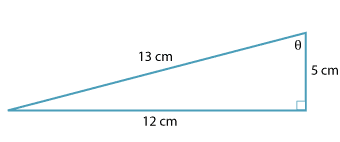#### Question 1

For the triangle pictured.Detailed description

a b sin $$\theta$$ = numerator— denominator cos $$\theta$$ = numerator— denominator tan $$\theta$$ = numerator—  denominator